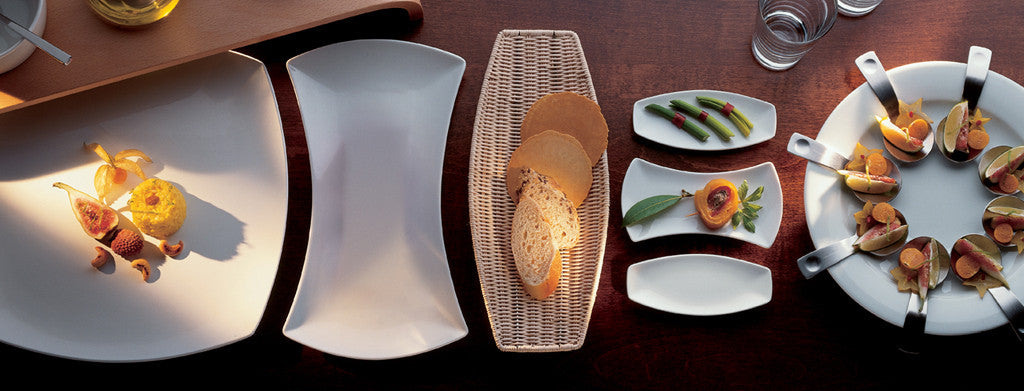Shopping Cart

### Refine

View all

#### Price# Crockery£7.08 each (ex VAT)£13.06 each (ex VAT)£10.48 each (ex VAT)£15.08 each (ex VAT)£12.10 each (ex VAT)£10.30 each (ex VAT)£10.52 each (ex VAT)£9.68 each (ex VAT)£13.06 each (ex VAT)£10.48 each (ex VAT)£8.90 each (ex VAT)£9.10 each (ex VAT)£15.08 each (ex VAT)£12.10 each (ex VAT)£10.30 each (ex VAT)£10.52 each (ex VAT)£9.68 each (ex VAT)£7.08 each (ex VAT)£4.90 each (ex VAT)£6.48 each (ex VAT)£7.36 each (ex VAT)£3.74 each (ex VAT)£6.02 each (ex VAT)£4.90 each (ex VAT)£6.48 each (ex VAT)£5.80 each (ex VAT)£4.24 each (ex VAT)£7.36 each (ex VAT)£4.32 each (ex VAT)